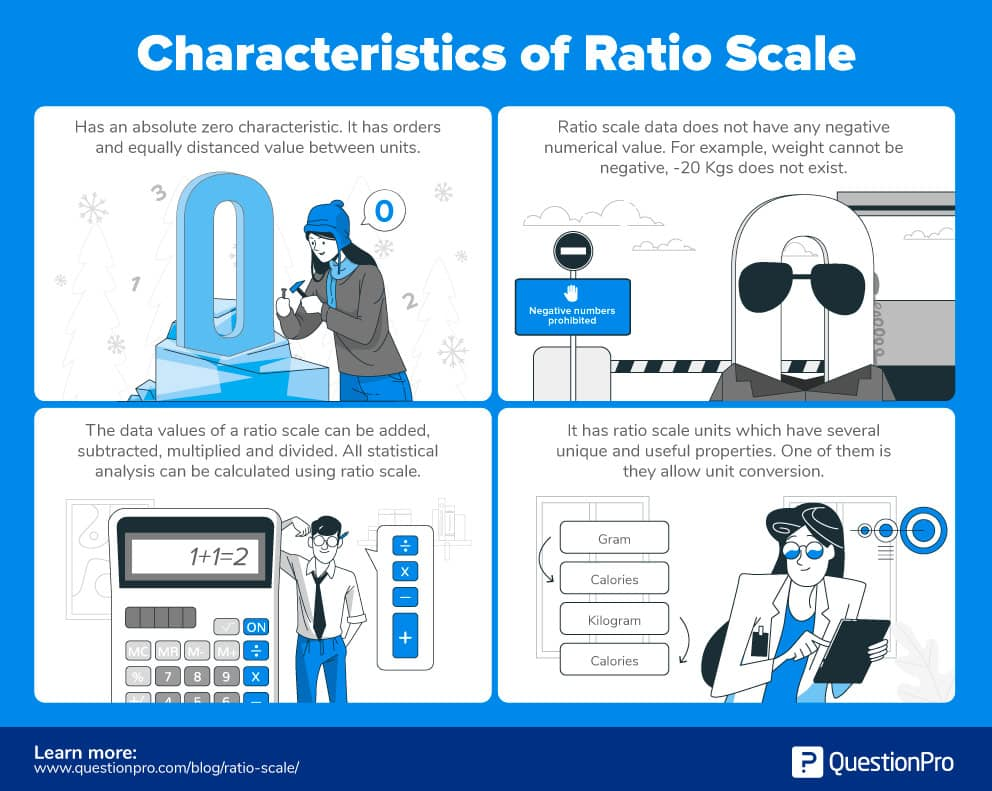# Ratio Scale: Definition, Characteristics and Examples

## The Definition

Ratio scale is a type of variable measurement scale which is quantitative in nature. It allows any researcher to compare the intervals or differences. Ratio scale is the 4th level of measurement and possesses a zero point or character of origin. This is a unique feature of this scale. For example, the temperature outside is 0-degree Celsius. 0 degree doesn’t mean it’s not hot or cold, it is a value.

Following example of ratio level of measurement to help understand the scale better.

Please select which age bracket do you fall in?

• Below 20 years
• 21-30 years
• 31-40 years
• 41-50 years
• 50 years and above

Ratio scale has most of the characteristics of the other three variable measurement scale i.e nominal, ordinal and interval. Nominal variables are used to “name,” or label a series of values. Ordinal scales provide a sufficiently good amount of information about the order of choices, such as one would be able to understand from using a customer satisfaction survey. Interval scales give us the order of values and also about the ability to quantify the difference between each one. Ratio scale helps to understand the ultimate-order, interval, values, and the true zero characteristic is an essential factor in calculating ratios.

A ratio scale is the most informative scale as it tends to tell about the order and number of the object between the values of the scale. The most common examples of this scale are height, money, age, weight etc. With respect to market research, the common examples that are observed are sales, price, number of customers, market share etc.

### Characteristics of Ratio Scale

1. Ratio scale, as mentioned earlier has an absolute zero characteristic. It has orders and equally distanced value between units. The zero point characteristic makes it relevant or meaningful to say, “one object has twice the length of the other” or “is twice as long.”
1. Ratio scale doesn’t have a negative number, unlike interval scale because of the absolute zero or zero point characteristic. To measure any object on a this scale, researchers must first see if the object meets all the criteria for interval scale plus has an absolute zero characteristic.
1. Ratio scale provides unique possibilities for statistical analysis. In this scale, variables can be systematically added, subtracted, multiplied and divided (ratio). All statistical analysis including mean, mode, the median can be calculated using it. Also, chi-square can be calculated on this scale variable.
1. Ratio scale has units which have several unique and useful properties. One of them is they allow unit conversion. Take an example of calculation of energy flow. Several units of energy occur like Joules, gram-calories, kilogram-calories, British thermal units. Still more units of energy per unit time (power) exist kilocalories per day, liters of oxygen per hour, ergs, and Watts.### Scale Examples

The following are the most commonly used examples:

1. What is your height in feet and inches?

• Less than 5 feet.
• 5 feet 1 inch – 5 feet 5 inches
• 5 feet 6 inches- 6 feet
• More than 6 feet

2.  What is your weight in kgs?

• Less than 50 kgs
• 51- 70 kgs
• 71- 90 kgs
• 91-110 kgs
• More than 110 kgs

3. How much time do you spend daily watching television?

• Less than 2 hours
• 3-4 hours
• 4-5 hours
• 5-6 hours
• More than 6 hours

Let us take the example of speed to understand this scale better.

Physics defines speed as speed is the distance traveled per unit time. Here the unit for time can be kept constant and a unit for distance traveled can vary, for example, can be in kilometers or miles which means 20 km/hour is equivalent to 12.43 mph.

If you have seen cars in Canada, the speedometer in the car has both miles and km on it.